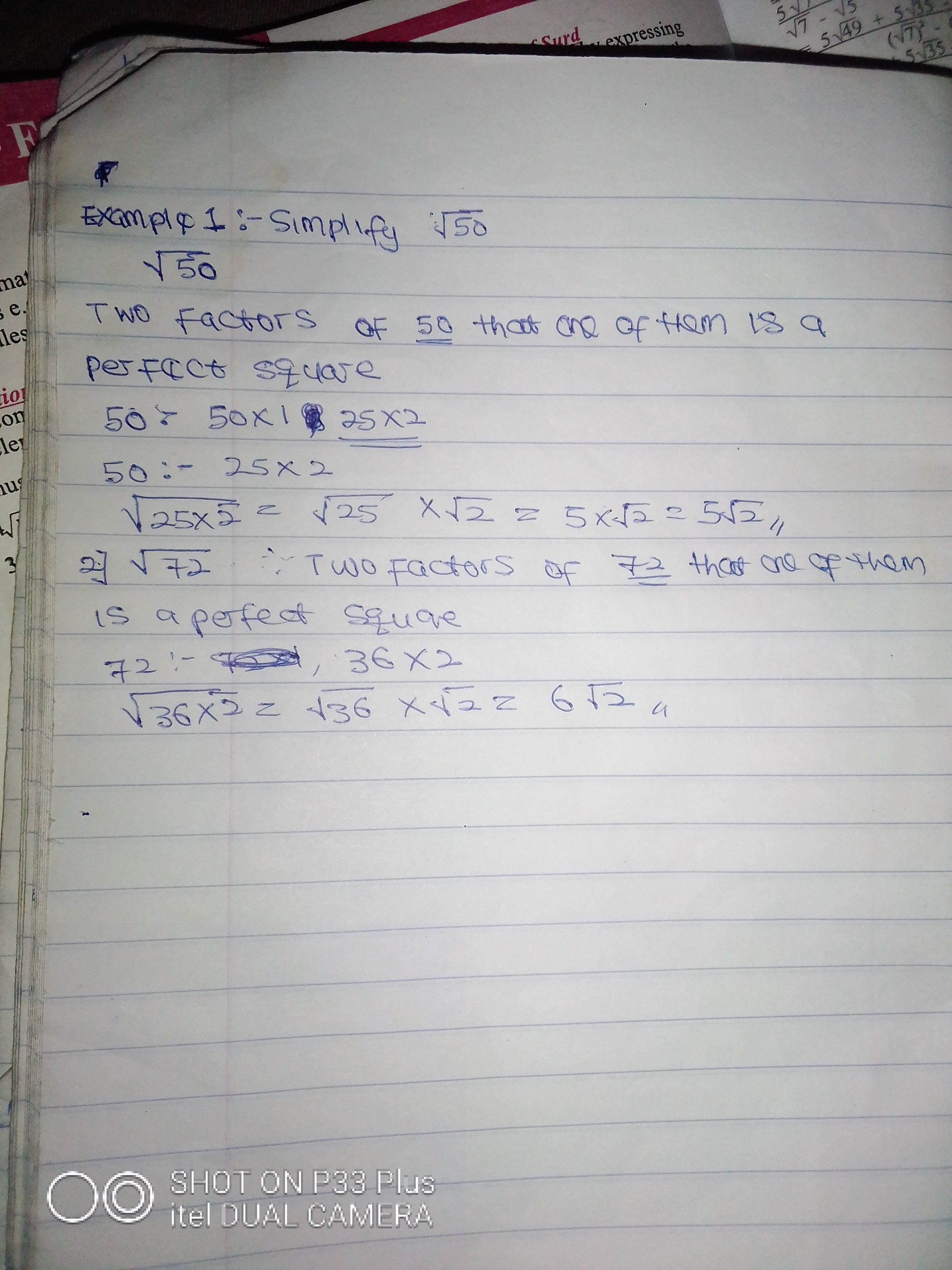Sign inEducation

College Education

# Mathematics Is Easy: See This Simple Mathematics You Can Solve Without CalculatorGood evening to my dear readers, I know many people seeing this are the ones that believe in the power of calculation.

During this pandemic many states don't allow the students to go to school but they used online lectures as an alternative to teach students. I like to help students especially in a field of calculation, so am going to teach you mathematics today.I don't like hearing mathematics is hard, you just have to follow the usual ways, listening to the lectures, nothing is hard.

You don't need calculator to solve this type of mathematics.

Today's Topic is surd.What is a surd?

A surd is a mathematics of root members or elements or we can say it is an irrational numbers e.g √2, 2√3 and 8√3.

We have addition, subtraction, multiplication and division of surd.

Let talk about general simplification of surd.

Perfect square, square root and factors are really essential, for examplesIf you check the first question, I need to find two factors of 50 and one must be a perfect square. Do you know the meaning of perfect square. Perfect square are numbers after they are square root, it gives number without decimal point.

√9=3

√16=4

√25 =5

√36= 6

√81= 9

All of them are perfect square.

Let me solve a complex question on it.This addition and subtraction of surd, Continue on multiplication and division of surd in the next article.I think you really understand it, that is for today, ask questions below and I give you a clear answer. Follow me to get more questions on surd, let punish mathematics together.

Content created and supplied by: AMEBOIBADAN (via Opera News )

Mathematics Is Easy